• MATLABC 代码生成可用于称为 Embedded MATLAB:registered: 的 MATLAB 语言子集，该子集由 310 多个 MATLAB 运算符和函数、110 个定点工具箱函数和 35 个信号处理工具箱函数和新系统对象组成在信号处理模块组和...matlab
• Matlab分享系列 - 3 - Matlab_Simulink代码生成和优化 a. 嵌入式代码生成介绍 b. 演示代码生成及配置参数/信号/模块名/函数名 c. 模型与数据分离配置 d. 数据字典 e. 示例与实践 f. 代码生成向导使用 g. 更多自定义...
• MATLABCoder? 可以从MATLAB?代码生成独立的、可读性强、可移植的C /C++代码MATLAB Coder 支持程序控制结构，函数和矩阵操作。它可以生成MEX函数，该函数可以加速MATLAB代码的运行速度并且验证生成代码...模型生成C...

单独执行

与其他软件集成

加速仿真MATLAB算法

实现嵌入式系统

特点：

生成符合ANSI/ISO标准的C / C++ 代码；

可以对定点和浮点数学运算生成MEX函数；

可以利用工程管理工具来确定输入点和输入数据属性以及代码生成的配置选项；

可为变大小的数据提供静态或动态内存存储位置；

代码生成支持Communications System Toolbox?, DSP System Toolbox?, 和Computer Vision System Toolbox?许多函数和系统对象；

支持一般的MATLAB语言行为，包括矩阵操作，下标操作，程序控制语句(if，switch，for，while)以及结构体。MATLAB Coder  工程管理界面(左)和代码生成报告(右)，该报告显示了生成的C代码.

强大的功能

■   可生成代码的MATLAB 语言子集

MATLAB Coder可以针对某些MATLAB 语言生成代码，这些语言一般都是设计工程师在开发大型系统的算法时经常用到的。这些支持的子集包括超过400个操作符和MATLAB中的函数。

MATLAB Coder支持对Aerospace Toolbox，Image Processing Toolbox?和Signal Processing Toolbox?中的部分函数生成代码，而且支持对Communications System Toolbox?，Computer Vision System Toolbox?和DSP System Toolbox?中的系统对象生成代码。

MATLAB Coder支持开发算法时用到的大部分MATLAB语言，包括：

矩阵和数组：比如持续变量，全局变量，矩阵操作，N维数组，下标操作和帧

类和数据类型：比如复数， 整形数据匹配，单精度双精度，定点运算，字符，结构体，数值类和变长度数据

编程结构：比如MATLAB程序控制结构(if, for, while, switch)和数学运算，逻辑关系操作符

函数：比如函数句柄，子函数和部分MATLAB函数，变长度输入和输出声明列表和子函数的调用

■MATLAB Coder的应用

MATLAB Coder的应用通过使用MATLAB Coder，设计工程师可以在MATLAB中开发算法，然后生成可读性强和可移植的C/C++代码，利用该代码可以：

将MATLAB算法生成在PC机上可以运行的可执行文件；

可以通过用自动生成的MATLAB可执行文件(MEX函数)替换MATLAB代码来加快仿真速度，MEX函数就是调用编译好的C/C++代码；

可以将MATLAB算法作为一个编译好的库集成到其他的软件中；

可以在嵌入式处理器中执行和验证MATLAB算法。MATLAB Coder应用情况

■从MATLAB中生成代码

将MATLAB语言转换成C代码在执行过程中需要确定一定的要求，MATLAB Coder可以在MATLAB语言环境中指导你完成这个过程。由于MEX函数需要调用编译好的C代码，所以可以通过生成MEX函数，然后在MATLAB中运行该函数来确保你的算法符合代码生成规则。MATLAB    Coder 生成一个报告，这个报告中会指出在从MATLAB算法到生成代码过程中必须改正的错误。通过不断的修正错误、生成MEX函数这种迭代过程直到MATLAB算法满足代码生成的要求，这时就可以生成C/C++的源代码或者MEX函数了。

C 或者C++源代码可以用来：

将MATLAB算法生成在PC机上可以运行的可执行文件；

创建一个库；

作为嵌入式代码运行。

MEX文件可以代替MATLAB代码被调用：

可以在MATLAB中验证编译后的代码

加速执行速度通过使用MATLAB Coder从MATLAB生成代码的三步迭代流程Simulink中的雷达跟踪模型，这个模型使用MATLAB Function模块在这个模块中有用MATLAB编写的卡尔曼滤波算法

Embedded Coder可以针对支持的嵌入式处理器、面向目标的快速控制原型板，及微处理器的需要和微处理器生成代码，它提供了配置选项和可以更好的控制生成代码的函数、文件和数据的高级优化选项，因此它扩展了MATLAB Coder和Simulink Coder。Embedded Coder 提高了代码效率，并且能很方便的集成已有代码，数据类型和产品中的标定参数。

使用Embedded Coder生成的代码，可以导出到第三方的开发环境中，可以在嵌入式系统中自动创建可执行文件。生成的代码可以在处理器上执行用来验证性能，可以通过PIL仿真和代码剖析的方法来查看代码在硬件上的运行情况。

MATLAB Coder可以从MATLAB代码生成独立的、可读性强、可移植的C/C++代码。

使用MATLAB Coder产生代码的3个步骤：准备用于产生代码的MATLAB算法；检查MATLAB代码的兼容性(有些matlab代码语句并不能生成c/c++代码)；产生最终使用的源代码或MEX。

利用MATLAB Coder生成c++代码，并在vs2008中验证：

一个简单的例子，两数相乘：

1、安装matlab2011a或者更新版本；

2、简单生成一个foo.m文件；

function c = foo(a, b)%#codegen

%This function muliplies a and b

c = a * b

其中，%#codegen可以防止出现警告错误

3、在命令窗口，输入mex -setpu,选中一个存在的编译器；

4、在命令窗口输入coder(图形界面)，回车，弹出MATLAB Coder Project对话框；

5、在New选项卡Name中输入一个工程名foo.prj；点击Ok，弹出MATLAB Coder MEX Function对话框；

7、单击变量a,选择Define by Example…,弹出MATLAB Coder Define by Example对话框，在MATLAB Expression中输入5，点击OK；同样变量b也进行相应操作，输入6；

8、选中Build选项卡，Output type中选择c/c++ Static Library；选中Generate code only；

9、点击More settings，GeneralàLanguage选择C++；Interface选项中去掉所有选项；Close；

10、点击Build，进行编译；点击View report，弹出Code Generation Report对话框，此时，变量a、b、c会显示相应的变量信息；

11、利用codeblocks建立一个控制台应用程序，将生成的相关文件foo.h、foo.cpp、rtwtypes.h、foo_types.h等文件拷到相关目录下并添加到应用程序中；

12、在foo.cpp文件中添加#include “stdafx.h”；

13、test.cpp文件中代码为：

#include "stdafx.h"

#include "foo.h"

#include

using namespace std;

int _tmain(int argc, _TCHAR* argv[])

{

double a = 0.0, b = 0.0, c = 0.0;

cin>>a>>b;

c = foo(a, b);

cout<

return 0;

}

一个复杂的例子，求一个数的n次方根：

1、  两个.m文件：

nrt.m:

function [nth_rt, iterations, hstry] = nrt(varargin)%#codegen

%This function will use a Newton Search Technique to find

%the nth root of a number, a, to the tolerance, tol.

%The square root

% nrt(10, 2), or nrt(10, 2, 1e-9)

%The "n" root

%nrt(10, n), or nrt(10, n, 1e-9)

a = varargin{1};

n = varargin{2};

if nargin ~= 3

tol = 1e-9;

else

tol = varargin{3};

end

if a < 0

nth_rt = 0;

iterations = 0;

hstry = 0;

else

[nth_rt, hstry] = newtonSearchAlgorithm(a, n, tol);

iterations = length(find(hstry ~= 0));

%iterations = sum(hstry ~= 0);

end

newtonSearchAlgorithm.m：

function [x, h] = newtonSearchAlgorithm(b, n, tol) %#codegen

%Given, "a", this function finds the nth root of a

%number by finding where: x^n-a = 0

coder.inline('never'); %使其生成一个单独的c++文件

notDone = 1;

aNew    = 0; %Refined Guess Initialization

a       = 1; %Initial Guess

cnt     = 0;

h = zeros(50, 1);

h(1)    = a;

while notDone

cnt = cnt + 1;

[curVal, slope] = f_and_df(a, b, n); %  square

yint = curVal - slope * a;

aNew = -yint / slope; %The new guess

h(cnt) = aNew;

if (abs(aNew-a) < tol) %Break if it's converged

notDone = 0;

elseif cnt > 49 %after 50 iterations, stop

notDone = 0;

aNew = 0;

else

a = aNew;

end

end

x = aNew;

function [f, df] = f_and_df(a, b, n)

%Our function is f=a^n-b and it's derivative is n*a^(n-1).

f  = a^n-b;

df = n*a^(n-1);

2、  在命令窗口输入coder(图形界面)，回车，弹出MATLAB Coder Project对话框；

3、在New选项卡Name中输入一个工程名nrt.prj；点击Ok，弹出MATLAB Coder MEX Function对话框；

5、添加三个输入，分别为10、2、1e-9；两个输入也可以；

6、选中Build选项卡，Output type中选择c/c++ Static Library；选中Generate code only；

7、点击More settings，General-->Language选择C++；Interface选项中去掉所有选项；Close；

8、点击Build，进行编译；点击View report，弹出Code Generation Report对话框；

9、利用vs2008建立一个控制台应用程序，将生成的相关文件nrt.cpp、nrt.h、newtonSearchAlgorithm.cpp、newtonSearchAlgorithm.h、nrt_types.h、rtwtypes.h拷到相关目录下并添加到应用程序中；

10、分别在nrt.cpp、newtonSearchAlgorithm.cpp文件中添加#include “stdafx.h”；

11、test.cpp文件中代码为：

#include "stdafx.h"

#include "nrt.h"

#include

using namespace std;

int _tmain(int argc, _TCHAR* argv[])

{

double varargin_1 = 0, varargin_2 = 0, varargin_3 = 1e-9;

cin>>varargin_1>>varargin_2;

double nth_rt = 0, iterations = 0;

double hstry_data = {0};

int hstry_sizes = {0};

nrt(varargin_1, varargin_2, varargin_3, &nth_rt, &iterations, hstry_data, hstry_sizes);

cout<

cout<

cout<

for (int i=0; i<50; i++)

{

cout

cout<

return 0;

}

展开全文• 将matlab代码转换成C代码

万次阅读 2018-11-29 22:47:15
MATLAB Coder可以从MATLAB代码生成独立的、可读性强、可移植的C/C++代码。本文基于matlab2015a编制，但是也适用于matlab 2014~2016的版本。 1. 使用MATLAB Coder产生C代码的4个步骤： (1) 开发实现特定算法功能的...

MATLAB Coder可以从MATLAB代码生成独立的、可读性强、可移植的C/C++代码。本文基于matlab2015a编制，但是也适用于matlab 2014~2016的版本。

1. 使用MATLAB Coder产生C代码的4个步骤：

(1) 开发实现特定算法功能的MATLAB代码及其测试文件；

(3) 产生最终使用的源代码或MEX;

(4) 利用MATLAB Coder生成c++代码，并在vs2008中验证。

2. Matlab功能模块代码及其测试代码

2.1 foo.m

function c = foo(a, b)%#codegen

%This function muliplies a and b

c = a.* b;

2.2 foo_high.m

其中，%#codegen可以防止出现警告错误

function r1 = foo_high(v1, v2)%#codegen

%This function muliplies a and b

r1=zeros(1,3);

r1(1)=foo(v1(1),v2(1));

r1(2:3) = foo(v1(2:3),v2(2:3));

2.3 test_main.m

clear all

clc

close all

a=8.97;

b=1.786;

a=[2.1 7.3 11];

b=[1.9 3.3 8];

rc = foo_high(a, b)

2.4 results

rc =3.9900 24.0900 88.0000

3. matlab代码转成C代码的详细步骤

(1) 在命令窗口，输入mex -setpu,选中一个存在的编译器；

(2) 在命令窗口输入coder(图形界面)，回车，弹出MATLAB Coder Project对话框；

或在matlab软件的界面中点击MatLab Coder图标。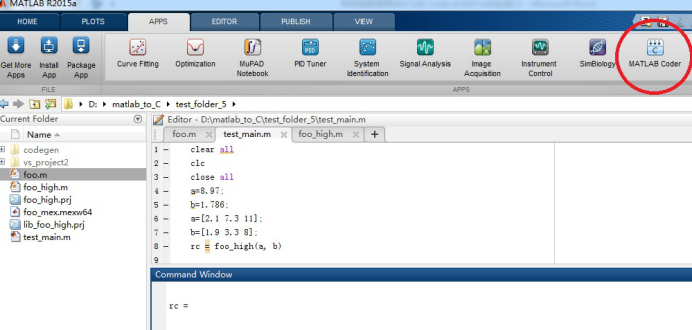生成的MATLAB Coder界面如下图所示：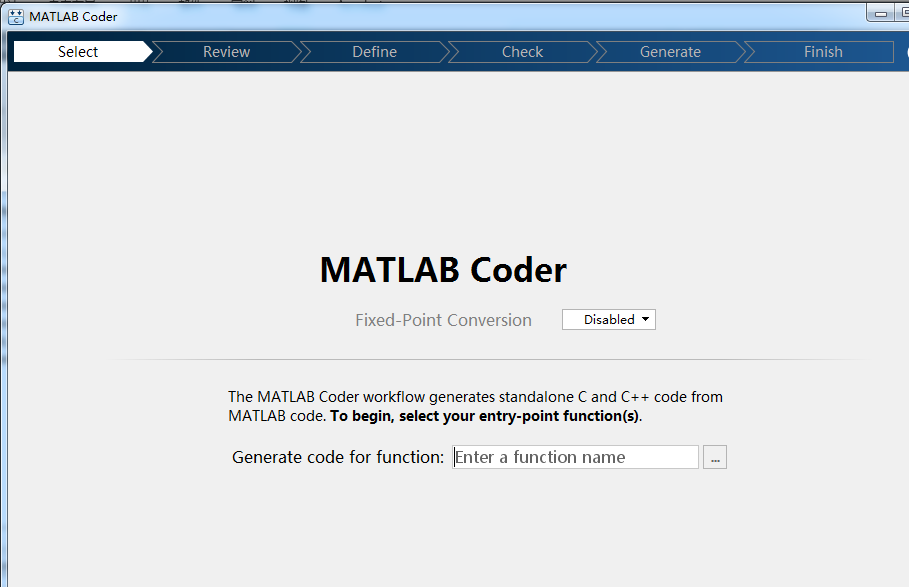(3) 在Generate code for function框中输入需要转换为C代码的matlab功能模块文件：foo.m和foo_high.m。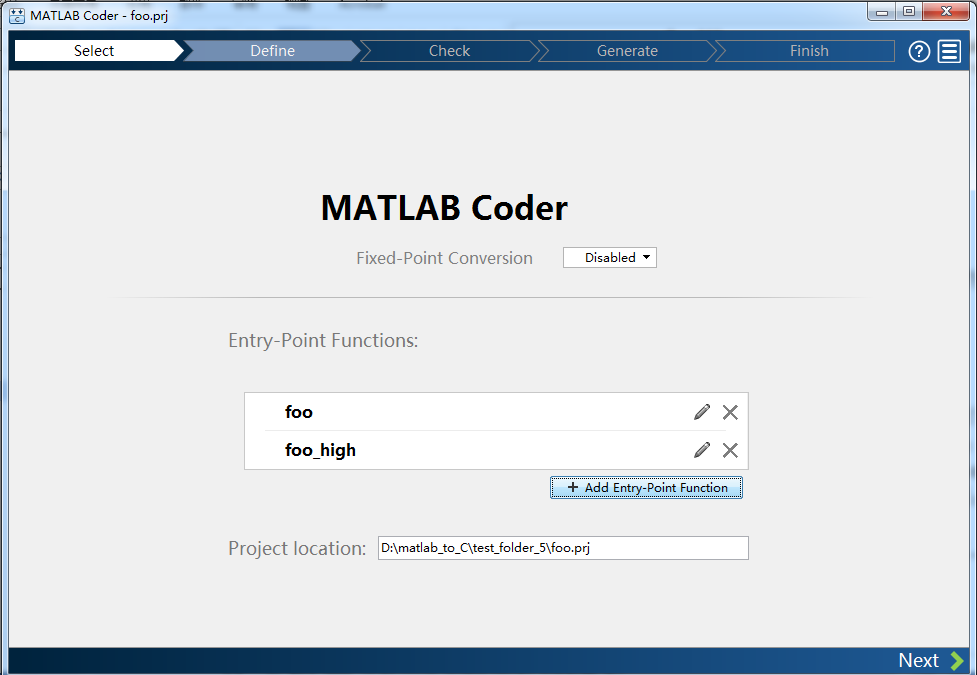(4) 点击next按钮，进入Define Input Types界面。输入test_main.m测试文件，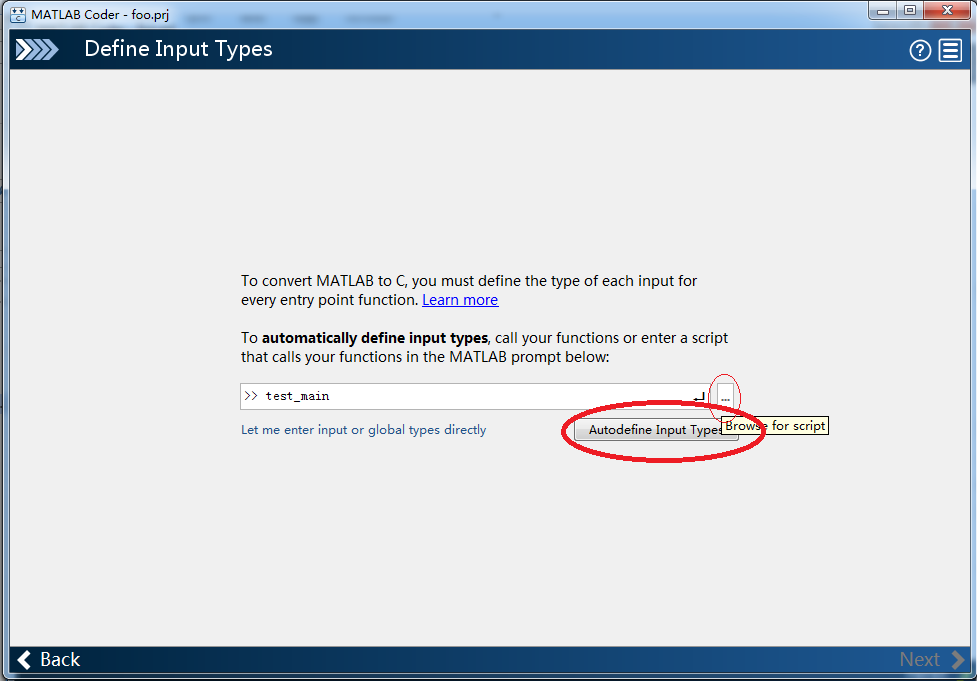(5) 点击Autodefine Input Types按钮，从而使得matlab能自动检测出foo函数的接口变量的维度和类型。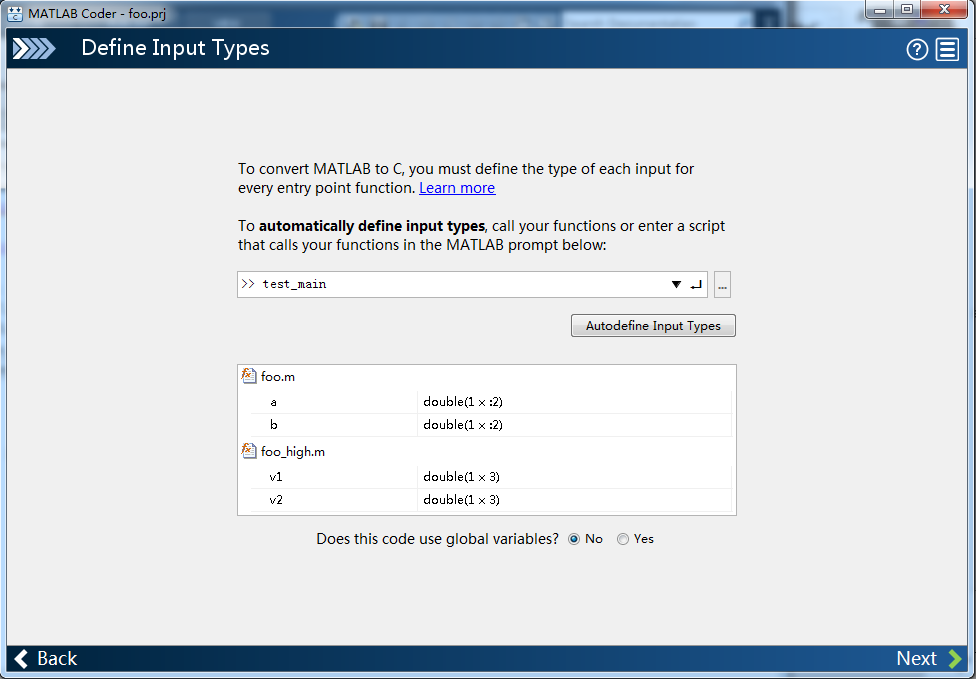(6) 点击next按钮，进入check for run-time issues界面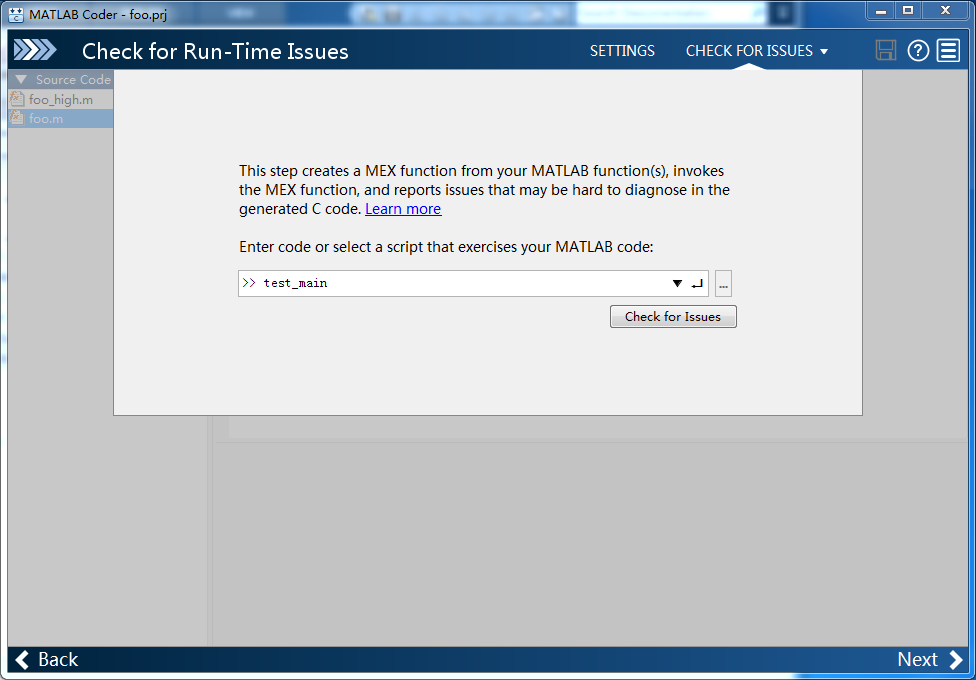点击check for issues按钮，进入对应的界面：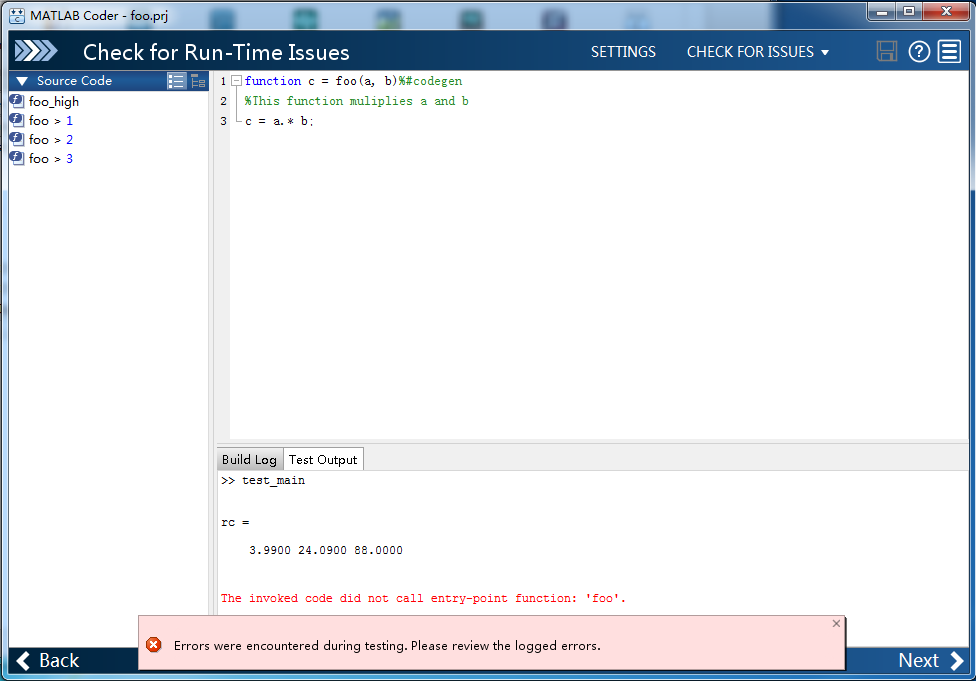上述问题表示被激活的代码没有调用foo函数的入口，即foo函数没有被调用。因为foo函数的功能已经被包含在foo_high函数中了。这里的红色部分可以不用理会。

(7) 点击next按钮，进入Generate Code界面：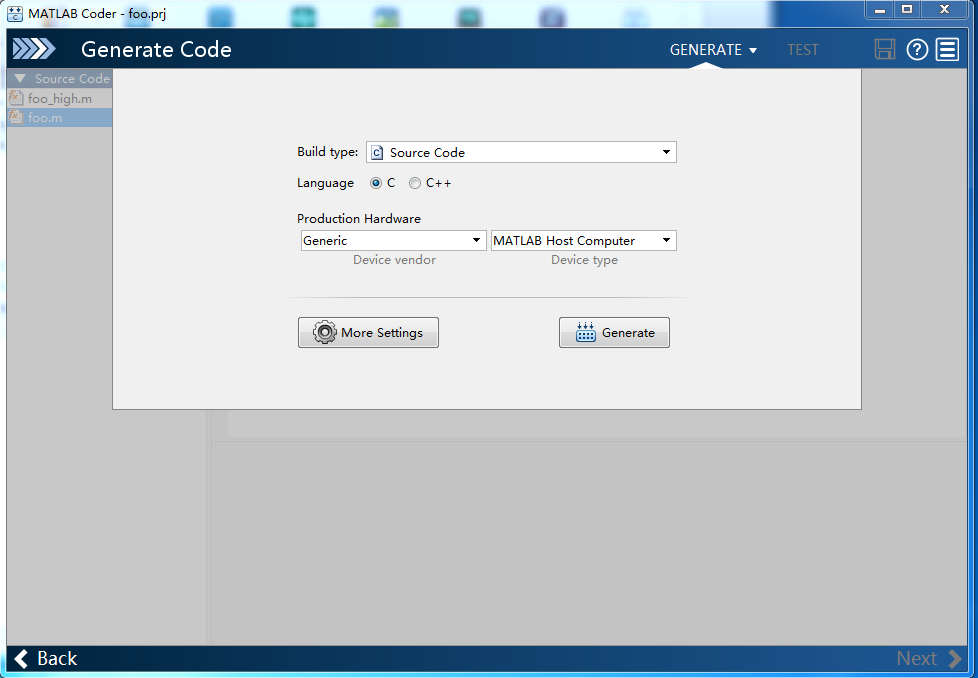点击“Generate”按钮，matlab生成对应的C代码并显示对应的界面：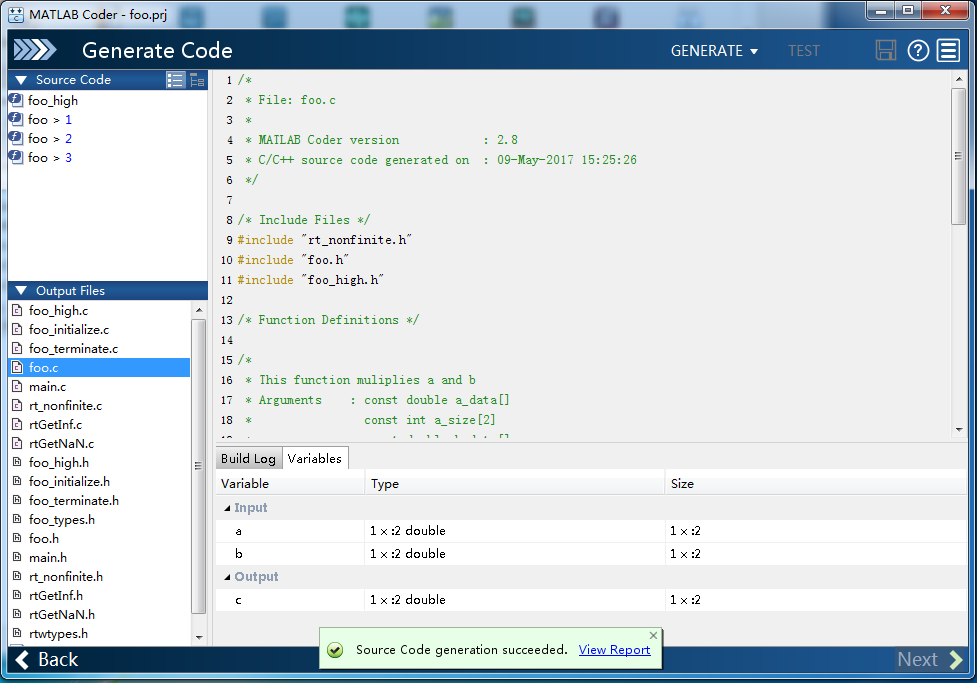(8) 点击next按钮，显示结束“Finish Workflow”界面：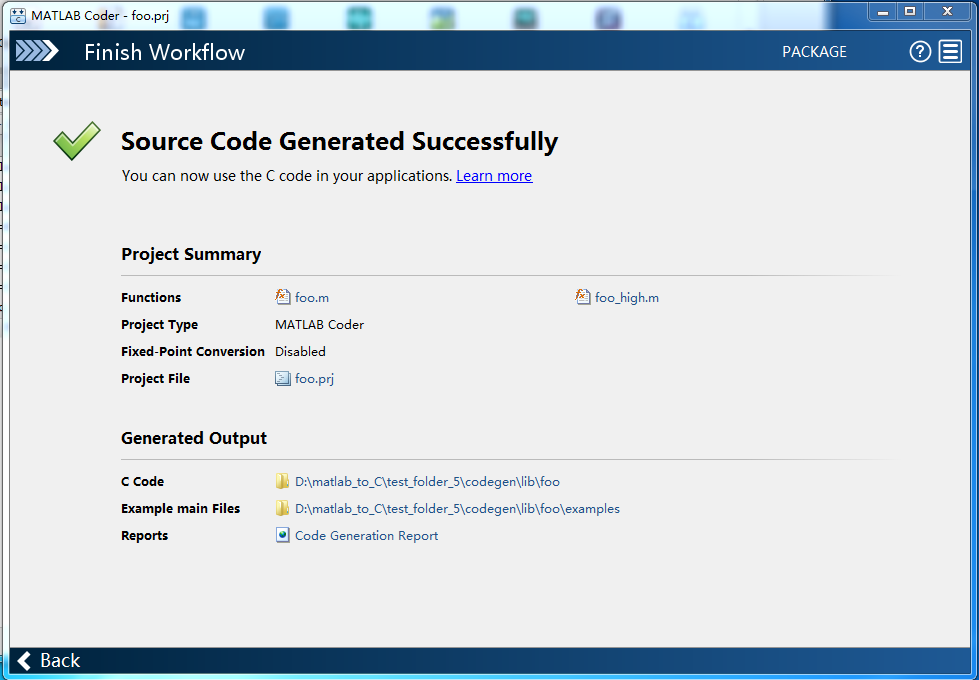4. 在vs2013中对转换获得的C代码进行测试。

Matlab在转换生成的C代码后，会生成一个codegen文件夹，下面包含两个子文件夹：lib和mex。Lib子文件夹下面包含xxx.h/xxx.c/xxx_initialize.c/xxx_initialize.c/xxx_tpyes.h

/rt_nonfinite.c/rt_nonfinite.h/rtGetInf.c/rtGetInf.h/rtGetNaN.c/rtGetNaN.h等文件，其中只有xxx.c是转换后所获得的核心文件。其它文件都是支持性文件。Mex文件夹下的文件是具有冗余的文件，可不予考虑。

4.1 foo_high.c

void foo_high(const double v1,const double v2,double r1)

{

int i1;

/* This function muliplies a and b */

r1 = v1 * v2;

/* This function muliplies a and b */

for (i1 = 0; i1 < 2; i1++) {

r1[1 + i1] = v1[1 + i1] * v2[1 + i1];

}

}

4.2 Main.c

#include <iostream>

#include <D:\matlab_to_C\test_folder_5\vs_project2\foo_high.c>

using namespace std;

int main()

{

double a = {4,9,2};

double b = {2,7,8};

double c;

//cin >> a >> b;

foo_high(a,b,c);

cout << "c = " << c << endl;

cout << "c = " << c << endl;

cout << "c = " << c << endl;

cout << "c = " << c << endl;

cin.get();

return 0;

}

5. Example of C_Plus_Plus code

5.1 func1

#include <iostream>

using namespace std;

int func1(int a, int b)

{

int i0;

i0 = a*b;

cout << "\n***Good Test*******" << endl;

//return(int)i0;

return i0;

}

5.2 main

#include <stdio.h>

#include <iostream>

extern int func1(int a, int b);

//#include"func1.cpp"

using namespace std;

int main(void){

int i, a;

int b = {11,22,33,44,55,66,77,88};

cout << "*****Good Test*******" << endl;

for (i = 0; i <= 9; i++)

a[i] = i;

for (i = 9; i >= 0; i--)

printf("%d ", a[i]);

for (i = 7; i >= 0; i--)

printf("%d ", b[i]);

int rt;

rt = func1(8, 9);

cout << "rt="<< rt << endl;;

cin.get();

return 0;

}

7. 备注

(2) 单击变量a,选择Define by Example…,弹出MATLAB Coder Define by Example对话框，在MATLAB Expression中输入5，点击OK；同样变量b也进行相应操作，输入6；

(3) 选中Build选项卡，Output type中选择c/c++ Static Library；选中Generate code only；

(4) 点击More settings，GeneralàLanguage选择C++；Interface选项中去掉所有选项；Close；

(5) 点击Build，进行编译；点击View report，弹出Code Generation Report对话框，此时，变量a、b、c会显示相应的变量信息；

(6) 利用vs2008建立一个控制台应用程序，将生成的相关文件foo.h、foo.cpp、rtwtypes.h、foo_types.h拷到相关目录下并添加到应用程序中；

(7) 在foo.cpp文件中添加#include “stdafx.h”；

(8) test.cpp文件中代码为：

#include "stdafx.h"

#include "foo.h"

#include <iostream>

using namespace std;

int _tmain(int argc, _TCHAR* argv[])

{ double a = 0.0, b = 0.0, c = 0.0;

cin>>a>>b;

c = foo(a, b);

cout<<"c = "<<c<<endl;

return 0;}

7. 一个复杂的例子

求一个数的n次方根：

4.1两个.m文件：

nrt.m:

function [nth_rt, iterations, hstry] = nrt(varargin)%#codegen

%This function will use a Newton Search Technique to find

%the nth root of a number, a, to the tolerance, tol.

%The square root

% nrt(10, 2), or nrt(10, 2, 1e-9)

%The "n" root

%nrt(10, n), or nrt(10, n, 1e-9)

a = varargin{1};

n = varargin{2};

if nargin ~= 3

tol = 1e-9;

else

tol = varargin{3};

end

if a < 0

nth_rt = 0;

iterations = 0;

hstry = 0;

else

[nth_rt, hstry] = newtonSearchAlgorithm(a, n, tol);

iterations = length(find(hstry ~= 0));

%iterations = sum(hstry ~= 0);

end

newtonSearchAlgorithm.m

function [x, h] = newtonSearchAlgorithm(b, n, tol) %#codegen

%Given, "a", this function finds the nth root of a

%number by finding where: x^n-a = 0

coder.inline('never'); %使其生成一个单独的c++文件

notDone = 1;

aNew = 0; %Refined Guess Initialization

a = 1; %Initial Guess

cnt = 0;

h = zeros(50, 1);

h(1) = a;

while notDone

cnt = cnt + 1;

[curVal, slope] = f_and_df(a, b, n); % square

yint = curVal - slope * a;

aNew = -yint / slope; %The new guess

h(cnt) = aNew;

if (abs(aNew-a) < tol) %Break if it's converged

notDone = 0;

elseif cnt > 49 %after 50 iterations, stop

notDone = 0;

aNew = 0;

else

a = aNew;

end

end

x = aNew;

function [f, df] = f_and_df(a, b, n)

%Our function is f=a^n-b and it's derivative is n*a^(n-1).

f = a^n-b;

df = n*a^(n-1);

2、 在命令窗口输入coder(图形界面)，回车，弹出MATLAB Coder Project对话框；

3、在New选项卡Name中输入一个工程名nrt.prj；点击Ok，弹出MATLAB Coder MEX Function对话框；

5、添加三个输入，分别为10、2、1e-9；两个输入也可以；

6、选中Build选项卡，Output type中选择c/c++ Static Library；选中Generate code only；

7、点击More settings，General-->Language选择C++；Interface选项中去掉所有选项；Close；

8、点击Build，进行编译；点击View report，弹出Code Generation Report对话框；

9、利用vs2008建立一个控制台应用程序，将生成的相关文件nrt.cpp、nrt.h、newtonSearchAlgorithm.cpp、newtonSearchAlgorithm.h、nrt_types.h、rtwtypes.h拷到相关目录下并添加到应用程序中；

10、分别在nrt.cpp、newtonSearchAlgorithm.cpp文件中添加#include “stdafx.h”；

11、test.cpp文件中代码为：

#include "stdafx.h"

#include "nrt.h"

#include <iostream>

using namespace std;

int _tmain(int argc, _TCHAR* argv[])

{

double varargin_1 = 0, varargin_2 = 0, varargin_3 = 1e-9;

cin>>varargin_1>>varargin_2;

double nth_rt = 0, iterations = 0;

double hstry_data = {0};

int hstry_sizes = {0};

nrt(varargin_1, varargin_2, varargin_3, &nth_rt, &iterations, hstry_data, hstry_sizes);

cout<<"nth_rt = "<<nth_rt<<endl;

cout<<"iterations = "<<iterations<<endl;

cout<<"hstry_data = "<<endl;

for (int i=0; i<50; i++)

{

cout<<hstry_data[i]<<endl;

}

cout<<"hstry_sizes = "<<hstry_sizes<<endl;

return 0;

}

感谢 [南极粥](https://me.csdn.net/zyqdragon)
展开全文MATLAB c
• matlab生成c代码编译失败目录 问题 一家报纸编辑正在研究过去几年中有关H1B（H-1B，H-1B1，E-3）签证申请处理的移民数据趋势，试图找出获得批准的H1B签证数量最多的职业和州。 她从美国劳工部及其部门获得了统计...
• ## 一、整体思路利用Matlab的simulink搭建模型生成C代码，通过stm32cubemx生成工程，最后在KEIL或者IAR等 工具 里面编译生成代码下载至MCU中执行。 本文以流水灯建立模型和简单使用定时器为例进行演示。二、开发环境...

## 一、整体思路

二、开发环境

1、安装Matlab

Matlab版本建议在2013B以上，本文使用Matlab 2016a。

2、安装STM32硬件支持包

下载地址(https://www.stmcu.org.cn/document/detail/index/id-216053) 安装完成后重启MATLAB。官网文档中提到，当前只支持F4和F3部分系列，F1系列生成代码后编译可能会报错，本文使用STM32-MAT版本V4.3.0。

3、安装STM32CUBEMX

用于配置单片机硬件资源，下载地址(https://www.stmcu.org.cn/document/detail/index/id-214984)

4、安装KEIL或者IAR等IDE

用于配置工程文件。

三、STM32硬件资源配置

1、打开STM32CUBEMX

2、选择STM32芯片

以STM32F103R6为例。如果搜索不到，那么可能需要下载安装库文件 下载对应的库文件即可。 搜索到芯片后，选中点击“Start Project”，进入配置界面。

3、首先选择时钟

这里选择HSE，使用外部晶振

4、再配置GPIO

选择PA1-5作为LED灯。引脚状态选择为输出。 再根据硬件设计，配置IO上拉还是下拉。

5、最后配置时钟

选择最大72MHz。

6、保存工程

输入工程文件信息 选择生成的工程文件，如果是KEIL，那么选择“MDK-ARM V5”。 最后保存即可。

1、配置MATLAB搜索路径

打开MATLAB，首先配置MATLAB搜索路径。 将STM32-MAT的安装路径添加进来。 添加完成后将MATLAB当前工作路径修改为第三步中IOC文件位置，默认是在Matlab安装位置，不修改的话后面生成代码的时候会报错。 最后建议重启一下MATLAB。

新建一个空白模型。 打开配置界面

3、选择STM32配置文件

选择stm32.tlc。 解算器(Solver)选项默认为固定步长，即“Fixed-step”，然后修改步长为1/10000，即0.1ms。也可以根据实际情况修改，默认为auto可能会导致程序运行时无法显示出效果。 添加代码注释

4、选择cubeMX路径

五、模型搭建

1、配置芯片

选择MCU config，将右侧模块拖动至刚刚新建的空白模型。 双击刚刚拖出来的模块，进入配置界面，选择第三步生成的IOC文件。

2、选择GPIO模块

3、模型设计

使用”Counter Free-Running”模块进行计数，参数配置如下 此配置的意思为每0.5秒计数一次，计数的最大值为2^Nbits – 1，其中Nbits为所填的2，所以这里最大值为3。 示波器显示和预期值一致。 然后通过一个关系比较符，当计数值分别等于0,1,2,3,时，相应的GPIO输出为1。 示波器仿真结果如图 最后模型如图所示

六、代码生成

模型设计完成后，仿真结果无误，就可以进行代码生成。按“Ctrl+B”或者如图所示点击，进行代码生成。 最后生成工程目录如下 “stmDemo_stm32”目录为matlab生成的原始代码。 IOC文件为stm32cubemx工程文件。 Slx文件为simulink模型文件。 其他的为KEIL工程中的代码。

七、编译下载验证

1、仿真验证

打开工程，代码编译完成后，下载程序到目标板。 这里使用proteus进行仿真，仿真中流水灯切换速度为5秒，比预定的时间0.5秒慢了10倍，仿真提示” Simulation is not running in real time due to excessive CPU load.”，说明仿真时单片机运行速度不是实时的。 为了进一步验证，再使用一个定时器进行让LED5以1Hz的频率闪烁。配置如下： 模型如下：

2、开发板验证

最后以F429单片机为例，重新生成代码下载到单片机进行验证。IOC文件中，时钟配置为180MHz，其他步骤同第三~六步。 代码生成完成后对工程进行配置，设置程序下载完成后重置运行，下载器根据实际情况设置为”CMSIS-DAP-Debugger”。 编译完成下载至单片机中，看是否满足设计要求。 经过验证该代码无误。

八、注意事项

1、代码生成后的处理

生成代码后，由于使用了systick(系统定时器)，但是生成的代码中没有对其进行调用，所以需要在stm32f4xx_it.C中手动添加一行代码，否则程序可能无法正常运行。添加的代码为：HAL_SYSTICK_IRQHandler(); 该问题具体原因未知，可能和stm32cubemx配置或者版本有关。

2、Matlab工作路径

建议生成代码之前，将matlab工作路径指定到IOC文件位置，同时将SLX文件也放在同一个文件夹下，这样方便工程管理。

3、参考文档

在STM32-MAT安装路径下还有更多示例以及使用说明。

4、Invalid index问题

Invalid index. Component:Simulink | Category:Block diagram error

九、小结

总的来说该方法生成底层代码较复杂，而且配置繁琐。当然优点是基本不需要修改生成的代码，但实际上未能节省太多时间。

展开全文• matlab生成c代码缺陷热像仪的自动数据处理 一个使用MATLAB和Python的热成像数据处理程序，只需稍加手动即可从RAW数据生成APTC映射，二进制映射，置信度映射和深度3D模型。 创建zip文件并发送到服务器以在Hololens中...
• matlab生成c代码编译失败欢迎来到模块化跟踪框架（MTF）的家！ 介绍 MTF是基于的高效且可扩展的库，也称为。 它在此领域中利用跟踪器的模块化分解，其中每个跟踪器包括以下3个模块： 搜索方法（SM） ： ，，，，，...

替换过程

在示例模型中，您可以将 PI 控制器中的 Lookup 模块(查找表)替换为对一个现有 C 函数的调用。该函数在文件 SimpleTable.c 和 SimpleTable.h 中定义。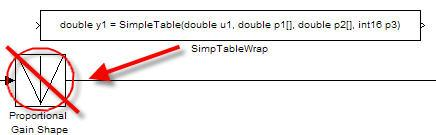创建调用 C 函数的模块

要指定对现有 C 函数的调用，请使用 S-Function 模块。您可以使用代码继承工具自动创建 S-Function 模块。在该工具中，您将首先为现有 C 函数指定接口。然后，工具使用该接口创建一个 S-Function 模块。

使用代码继承工具为 SimpleTable.c 中的现有 C 函数创建一个 S-Function 模块。

def = legacy_code('initialize')

您可以使用结构体 def 定义现有 C 代码的函数接口。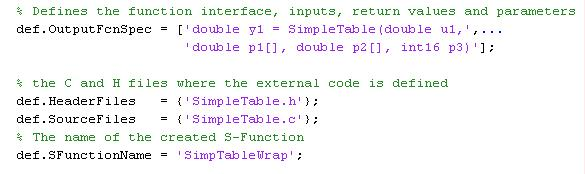legacy_code('sfcn_cmex_generate',def)

legacy_code('compile',def)

legacy_code('slblock_generate',def)

生成的 S-Function 模块调用 SimpleTable.c 中的 C 函数。您现在可以在模型中使用此 S-Function 模块。

legacy_code('sfcn_tlc_generate',def)

此命令创建一个 TLC 文件，该文件是 S-Function 的一个组件，指定如何为模块生成代码。

通过仿真验证外部代码

在 Simulink® 模型中集成现有 C 代码时，将验证生成的 S-Function 模块。

要验证 Lookup 模块的替换，请将 Lookup 模块产生的仿真结果与新 S-Function 模块产生的结果进行比较。

Sine Wave 模块从 [-2 :2] 产生输出值。

查找表的输入范围来自 [-1 :1]。

查找表输出输入的绝对值。

查找表根据输入范围对输出进行裁剪。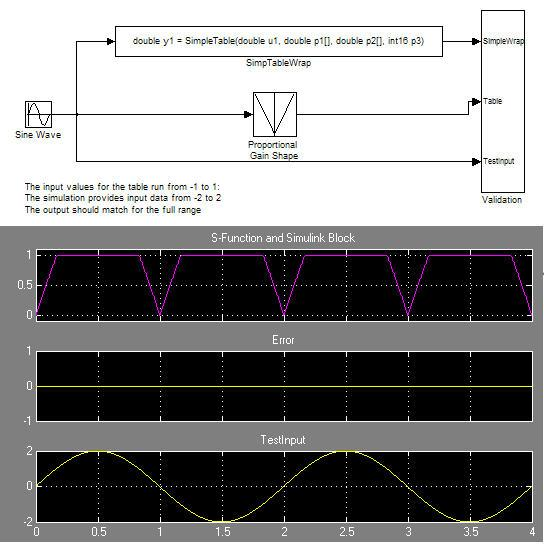将现有 C 函数代码作为独立组件进行验证后，在模型中验证 S-Function。要完成验证，请使用测试框架模型。

仿真结果与黄金值匹配。从生成的代码调用 C 函数

代码生成器使用 TLC 文件处理 S-Function 模块，就像处理任何其他模块一样。代码生成器可以使用 S-Function 模块实现表达式折叠，这是一种将多个计算合并到一个输出计算中的操作。

生成的代码现在调用 SimpleTable C 函数。

下面的图显示生成的代码在 C 代码集成之前和之后的情况。在集成之前，代码调用生成的查找例程。在集成之后，生成的代码调用 C 函数 SimpleTable。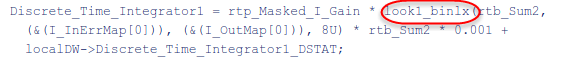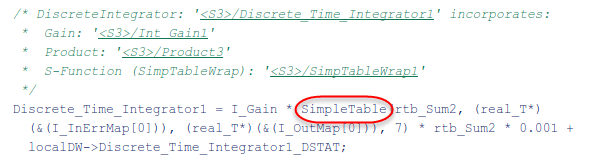展开全文• matlab生成c代码编译失败QCML：二次圆锥建模语言 这是给谁用的？ 对于凸优化的临时用户而言，该项目是更友好的用户体验，我们建议所有初学者从此处开始。 该项目主要是为想要部署优化代码的开发人员设计的。 它...
• 一、代码生成后，查看生成报告。如下图所示：代码文件简介：1、ert_main.c该文件中会调用 “模型名称_step();”函数，用于单步执行，可以根据实际情况放在定时器中断或者任务调度里面执行该函数。除此之外，该文件中...
• 该模型支持使用 simulink 模块为 texas Instruments C2000 微控制器生成代码。 这是使用 TMS320F28027 微控制器对感应电机进行速度控制的测试文件。matlab
• 本文主要以matlab 2019b版本，主要讲解怎么用simulink和fixed point tool生成嵌入式定点c代码： 在嵌入式代码设计中，由于浮点运算特别占用CPU资源，我们通常使用将浮点转化成定点再运算。下面讲用fixed point tool...嵌入式 matlab
• matlab生成c代码编译失败凝块 这只是令人惊叹的的npm发行。 之所以创建此软件包，是因为我认为cloc很棒，但是不想下载文件并将其提交到我的项目中。 安装及要求 要求 Perl cloc用perl编写，此程序包公开的二进制...
• 为模型生成 C 代码要从 Simulink® 模型、Stateflow® 图和 MATLAB® 函数生成 C 或 C++ 代码，请使用 Simulink Coder™ 产品。将生成代码用于仿真加速、快速原型和硬件在环 (HIL) 仿真等应用中。如果您刚开始使用...
• floating is out of range 以及神秘变MATLAB 代码自动生成(一) floating is out of range 以及神秘变量scale = 3.3121686421112381E-170在使用机器人最小惯性参数集计算机器人的力矩时，使用MATLAB自动生成代码到TI...
• matlab的egde源代码AR Drone 2.0 MATLAB / Simulink目标 AR Drone 2.0 Target为Parrot AR Drone 2.0的Simulink模型提供自动代码生成支持。 提供的Simulink模块可让您读取AR Drone 2.0上的所有传感器和两个摄像头。 ...
• 关于 DSP 代码生成功能，在 Matlab2013 以后的版本中被取消了。如果想在 2013 以后的版本中使用这个功能，需要下载相关的硬件支持包。 具体操作如下： 点击 Matlab 右上角的附加功能中的获取硬件支持包。 ...Simulink DSP 代码生成 CCS
• 一、代码生成后，查看生成报告。 如下图所示： [外链图片转存失败,站可能有防盗链机制,建议将图片保存下来直接上传(img-E99WyoQb-1576656105668)(https://img.rayu.me/2019/12/1127673928.png)] 代码文件简介： 1...matlab simulink
• Matlab生成stm32代码

万次阅读 多人点赞 2019-06-10 16:14:37matlab stm32 simulink
• 但是在硬件上应用的都是些代码，那有没有一种方法可以在matlab中直接生成代码，从而免去编写代码的麻烦呢?答案肯定是有的。 废话不多说，直接上图（自己弄得ppt，可能有不清楚的地方） 图片上有详细的解释，看不清的...matlab simulink 编程语言 算法 c语言
• Matlab代码生成fpga wurc_pcores HDL和MATLAB / System Generator模块用于数字信号处理。 实时增益控制模块与LMS6002D和wurc_fw项目集成在一起，用于兼容802.11ac的AGC。 LMS6002D无线电I / Q接口胶水，用于为数字预...

万次阅读 多人点赞 2019-09-07 11:12:44Simulink

千次阅读 多人点赞 2019-04-21 15:04:16
昨天参加了Mathworks公司在东南大学举办的关于MATLAB的培训，...嵌入式代码生成主要利用的是MATLAB中自带的MATLAB Coder模块MATLAB Coder可以从MATLABcode产生可读且可移植的C/C++程序，支持多数MATLAB语言和工具...

万次阅读 多人点赞 2019-02-27 19:29:32

万次阅读 多人点赞 2019-02-23 14:20:32MATLAB Simulink CCS DSP
• simulink生成的C/C++代码分析 模型生成C代码分析： 模型生成的代码主要包含三个部分： 1.首先第一个出现的函数是单步函数 step函数 2.第二个函数是初始化函数 initialize函数 3.第三个函数是终止函数 terminate函数matlab c++ simulink自动代码生成...

matlab模块生成c代码matlab 订阅